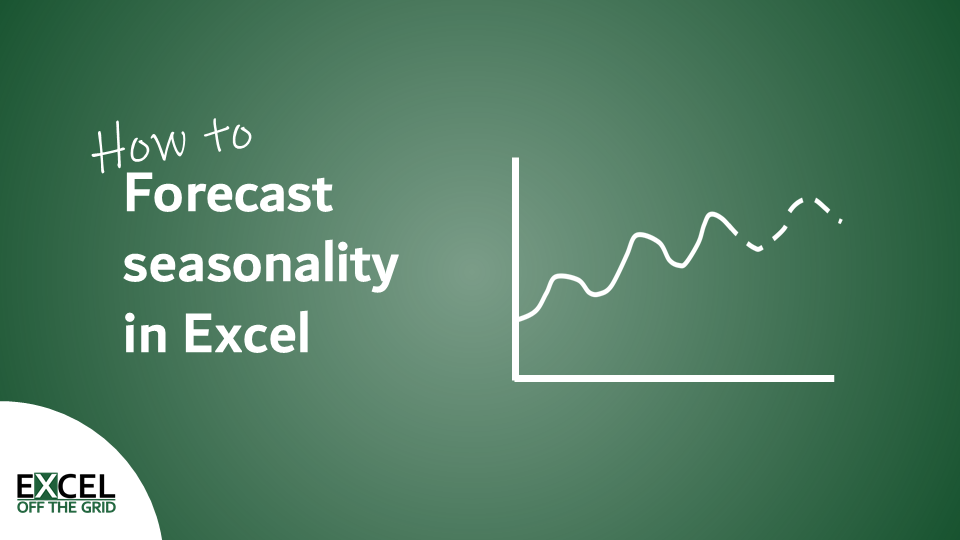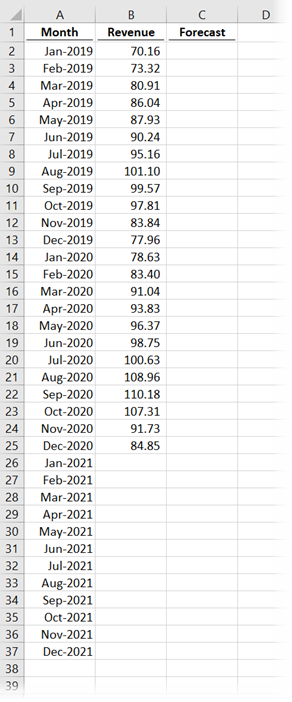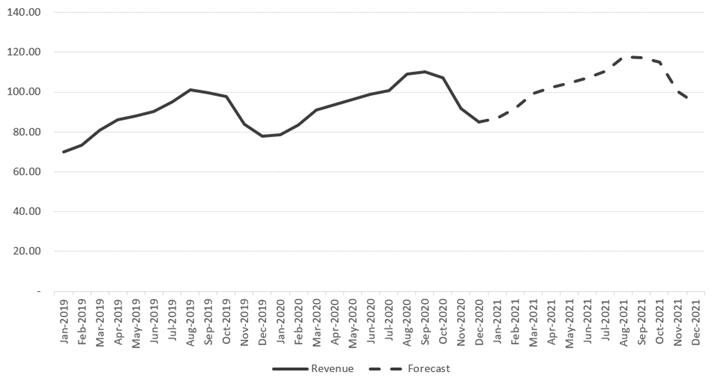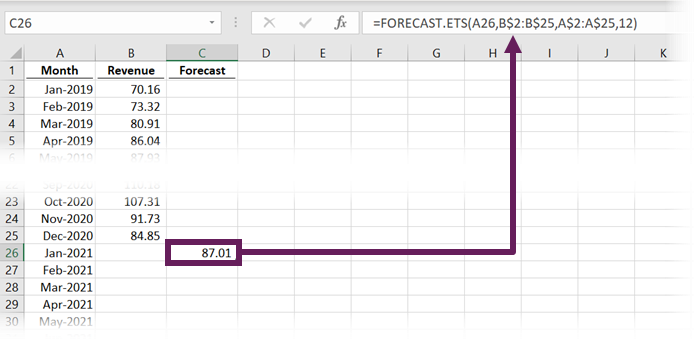# How to forecast seasonality in ExcelI have previously written about how to forecast seasonality in Excel using the FORECAST function.  However, with Excel 2016, Microsoft gave us an even easier method for forecasting seasonality.  Rather than using the FORECAST function with a lot of trickery, we can simply use the FORECAST.ETS function all by itself.

If you are using Excel 2013 or prior, check out my previous post here: https://exceloffthegrid.com/using-the-forecast-function-with-seasonality/

I recommend you download the example file for this post.  Then you’ll be able to work along with examples and see the solution in action, plus the file will be useful for future reference.

Contents

## The Scenario

The table below contains the data we will be using to demonstrate the function.There are 24 months of historical data.  Our goal is to create a forecast for the next 12 months which maintains the same seasonality pattern.

What you may not be able to see from the data above is that there is an underlying level of growth.  Therefore, not only is there seasonality but also a growth factor to consider.  The good news is that FORECAST.ETS makes this simple.

The final output we will create looks like this:The solid line is the historical values, and the dotted line is the forecast as calculated by FORECAST.ETS.

## The arguments of the FORECAST.ETS function

The FORECAST.ETS function on Excel calculates seasonal results using an exponential smoothing algorithm.  The syntax of the function is:

```=FORECAST.ETS(target_date, values, timeline, [seasonality],
[data_completion], [aggregation])```
• target_date: The date for which we want Excel to predict a value.  It can be a numeric or date/time value.
• values: The historical values to use as the basis for forecasting the future.
• timeline: The array or range of values representing the time over which the values occur. Any dates in the timeline must have a consistent step between them and can’t be zero.  It is not necessary for the timeline to be sorted, as Excel will automatically sort in memory before performing the calculation.
• [seasonality]: An optional value that represents the length of the seasonal pattern.
1 = Excel automatically detects the seasonal pattern (this is the default if not provided)
0 = Apply a linear calculation rather than a seasonal forecast
Any other whole number less than 8,764 = the length of the repeating pattern.
[data_completion]: An optional value of 1 or 0.  The function can work with up to 30% missing data.
1 = missing data is calculated as the average of the neighboring data points (the default, if argument not provided)
0 = missing data should be treated as zeros
• [aggregation]: An optional argument detailing how multiple values with the same time stamp should be treated.
1 = AVERAGE (the default, if argument not provided)
2 = COUNT
3 = COUNTA
4 = MAX
5 = MEDIAN
6 = MIN
7 = SUM

## Common errors

As a function, FORECAST.ETS can be susceptible to a lot of different types of errors.  It is doing some quite complex calculations; Excel can’t calculate a suitable value if we don’t use the correct inputs.  The most common errors are:

#VALUE!

• The fields target_date, seasonality, data_completion, or aggregation contain non-numeric values.
• The timeline contains duplicate values.

#N/A

• The values and timeline are not of the same length.

#NUM

• A consistent step cannot be found within the timeline.
• The value for seasonality is not a whole number between 0 and 8764
• The value for data_completion is not 0 or 1.
• The value for aggregation is not a whole number from 1 to 7.

Formula Magic with Dynamic ArraysOf all the features available in Excel dynamic arrays provide the most power for the smallest time investment.  Yet most Excel users do not even know what they are.

Have you ever faced these spreadsheet scenarios?

• How can I use VLOOKUP to return all the matching items, not just the first?
• How can I sort my information using a formula, so I don’t have to keep clicking the sort button?
• How can I quickly create unique lists of items to use with my SUMIFS calculation?
• How can I stop copying down formulas every time my source data changes.
• How can I build a PivotTable-like report, but using formulas so I don’t have to click refresh ever again.

Well, I’m here to give you some good news. with dynamic arrays, all these can be achieved easily 🙂

## Using the FORECAST.ETS function

OK, now we know how it works.  Let’s put it to the test.

If you’re working along with the example file, enter the following formula into cell C26:

`=FORECAST.ETS(A26,\$B\$2:\$B\$25,\$A\$2:\$A\$25,12)`• A26 – The date for which we want to calculate the value.
• B\$2:B\$25 – The range of previous values.
• A\$2:A\$25 – The dates of the previous values.
• 12 – The seasonality pattern is 12 because they are monthly values.

Now copy that formula down.  Provided you put your \$ symbols in the right place, you should get the following.Now let’s create a line chart to easily see the results in action:That is amazing.  Not only has Excel recognized the seasonality, but also the underlying growth rate.

I have added =NA() function into the blank cells so that they are not rendered on the chart (this is visible in the example file).

## How good is Excel at predicting the seasonal pattern?

Most of the time, we know how long our season is: quarterly, monthly, weekly, daily, etc.  But if we don’t, should we trust Excel to do it for us?  I have updated the function so that Excel automatically creates the seasonality argument…..

Oh dear!!! Maybe best to enter the seasonality value ourselves 🙂Get our FREE VBA eBook of the 30 most useful Excel VBA macros.
Automate Excel so that you can save time and stop doing the jobs a trained monkey could do.

Email Address * First Name *

Don’t forget: